Wave Velocities edit page

## Import an Elasticity Tensor

Let us start by importing the elastic stiffness tensor of an Olivine crystal in reference orientation from a file.

## Christoffel Tensor

The Christoffel Tensor is symmetric because of the symmetry of the elastic constants. The eigenvalues of the 3x3 Christoffel tensor are three positive values of the wave moduli which corresponds to \rho Vp^2 , \rho Vs1^2 and \rho Vs2^2 of the plane waves propagating in the direction n. The three eigenvectors of this tensor are then the polarization directions of the three waves. Because the Christoffel tensor is symmetric, the polarization vectors are perpendicular to each other.

## Elastic Wave Velocity

The Christoffel tensor is the basis for computing the direction dependent wave velocities of the p, s1, and s2 wave, as well as of the polarization directions. Therefore, we need also the density of the material, e.g.,

which we can write directly into the elastic stiffness tensor

the single crystal wave velocities are now computed by the command stiffnessTensor.velocity.html velocity>

As output we obtain three spherical functions vp, vs1 and vs2 representing the velocities of P, and fast and slow S-waves respectively in dependency of the propagation direction. The remaining three output variables pp, ps1, ps2 are spherical vector fields representing the polarization directions of these wave as functions of the propagation direction.

In order to visualize these quantities, there are several possibilities. Let us first plot the direction dependent wave speed of the p-wave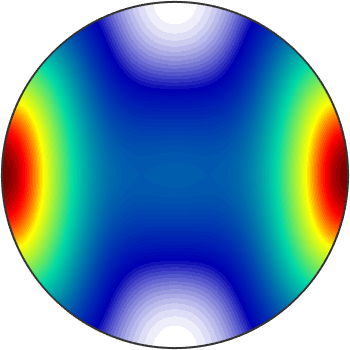Next, we plot on the top of this plot the p-wave polarization direction.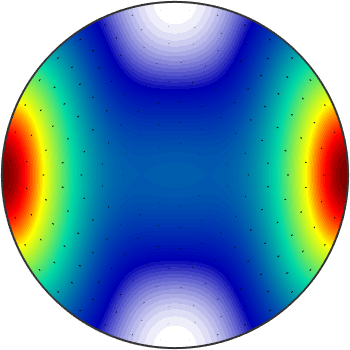We may even compute with these spherical functions as width ordinary values. E.g. to visualize the speed difference between the s1 and s2 waves we do.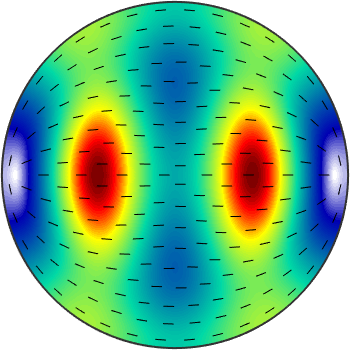When projected to a plane the different wave speeds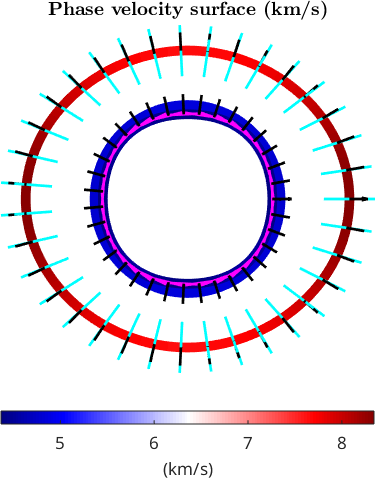Similarly, we can visualize the slowness surfaces (s/km)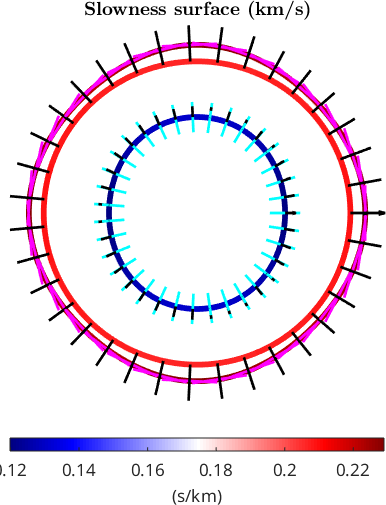set back default colormap